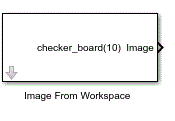# Image From Workspace

Import image from MATLAB workspace

• Library:
• Computer Vision Toolbox / Sources

•## Description

The Image From Workspace block imports an image from a matrix, 3-D array, MATLAB function, or variable stored in theMATLAB® workspace, to the Simulink® workspace.

## Ports

### Output

expand all

Output image, returned as a matrix or 3-D array. If the imported image is binary or grayscale image, the output is a matrix of size M-by-N. If the imported image is a color image, the output is a 3-D array of size M-by-N-by-3.

#### Dependencies

To enable this port ,set the Image signal parameter to `One multidimensional signal`.

Data Types: `single` | `double` | `int8` | `int16` | `int32` | `uint8` | `uint16` | `uint32` | `Boolean` | `fixed point`

Red color component of the image, returned as an M-by-N matrix. The block returns this output if the input is a color image. If the Image signal parameter is set to `Separate color signals` and the input image is binary or grayscale, the block returns only this port

#### Dependencies

To enable this port, set the Image signal parameter to `Separate color signals`.

Data Types: `single` | `double` | `int8` | `int16` | `int32` | `uint8` | `uint16` | `uint32` | `Boolean` | `fixed point`

Green color component of the image, returned as an M-by-N matrix. The block returns this output only if the input is a color image.

#### Dependencies

To enable this port, set the Image signal parameter to `Separate color signals`.

Data Types: `single` | `double` | `int8` | `int16` | `int32` | `uint8` | `uint16` | `uint32` | `Boolean` | `fixed point`

Blue color component of the image, returned as an M-by-N matrix. The block returns this output only if the input is a color image.

#### Dependencies

To enable this port ,set the Image signal parameter to `Separate color signals`.

Data Types: `single` | `double` | `int8` | `int16` | `int32` | `uint8` | `uint16` | `uint32` | `Boolean` | `fixed point`

## Parameters

expand all

Main

Specify the image as a vector, matrix, 3-D array, function, or MATLAB workspace variable. The value parameter can be:

• Vector — The value given to the Value parameter can be a row vector or column vector

• 3-D numeric array — The value given to the Value parameter can be 3-D array, which is generated using MATLAB functions

• MATLAB workspace variable — The value given to the Value parameter can be a variable name, which is MATLAB workspace variable

• MATLAB function — The value given to the Value parameter can be a MATLAB function with specified input arguments to generate a numeric array

This parameter specifies the sample time for block execution. Vector length must be 2 which represents [Period, Offset] values where offset should be less than period and non-negative. Given the default value of inf, the block outputs a matrix for grayscale and binary. The output is 3-D or 4-D, if the input image is a gray scale or color image respectively.

The value of the added dimension is equal to floor((Tsim/Ts)+1)

Tsim is the simulation time and Ts is the sample time, for block execution. For example, if the input image is a 256-by-256 matrix, the simulation time is 10 sec and the sample time is set to 0.5 sec, then the output is a 256-by-256-by-21 array.

Specify how the block returns the image read from the MATLAB workspace.

• `One multidimensional signal`— The block has one output port, Image, that returns the entire image.

• `Separate color signals` — The block has an output port for each color channel. Each port returns a single color channel of the image.

Specify labels for the output ports. Each label can be any value. Use the vertical bar character, |, as a delimiter to name all the output ports.

#### Dependencies

To enable this parameter, set the Image signal parameter to `Separate color signals`.

Data type

Specify the data type of the output image. If the data type of the imported image, specified in the Value parameter, differs from the value of the Output data type parameter, the block scales the pixel values, adds an offset to the pixel values so that they are in the dynamic range of the new data type, or does both. Double- and single-precision floating-point pixel values must be in the range of [0, 1].

Select this parameter if the input image data type is signed fixed-point.

#### Dependencies

To enable this parameter, set the Output data type parameter to `Fixed-point`.

Specify the word length, in bits, of the fixed-point output data type. The value should be less than 32.

#### Dependencies

To enable this parameter, set the Output data type parameter to `Fixed-point`.

Specify the scaling factor of the fixed-point output by either of the following two methods:

• `Best precision`— The output scaling automatically such that the output signal has the best possible precision.

• `User-defined` — The the output scaling in the Fraction length parameter.

#### Dependencies

To enable this parameter, set the Output data type parameter to `Fixed-point`.

Specify the number of bits to the right of the decimal point.

#### Dependencies

To enable this parameter, set the Output data type parameter to `Fixed-point`, and set the Set fraction length in output parameter to `User-defined`.

Specify any built-in or fixed-point data type. You can specify the below fixed-point data types using the Fixed-Point Designer™ library.

#### Dependencies

To enable this parameter, set the Output data type parameter to `User-defined`.

## Block Characteristics

 Data Types `Boolean` | `double` | `fixed point` | `integer` | `single` Multidimensional Signals `no` Variable-Size Signals `no`

## Extended Capabilities

### Functions

Introduced before R2006a